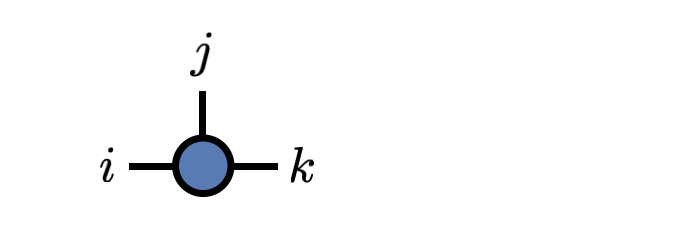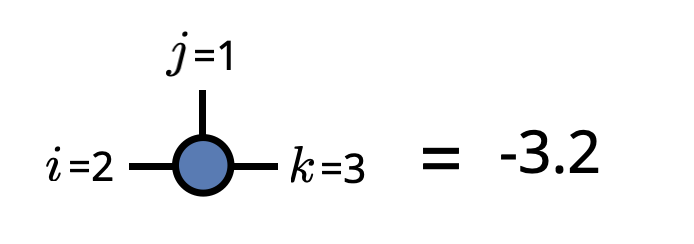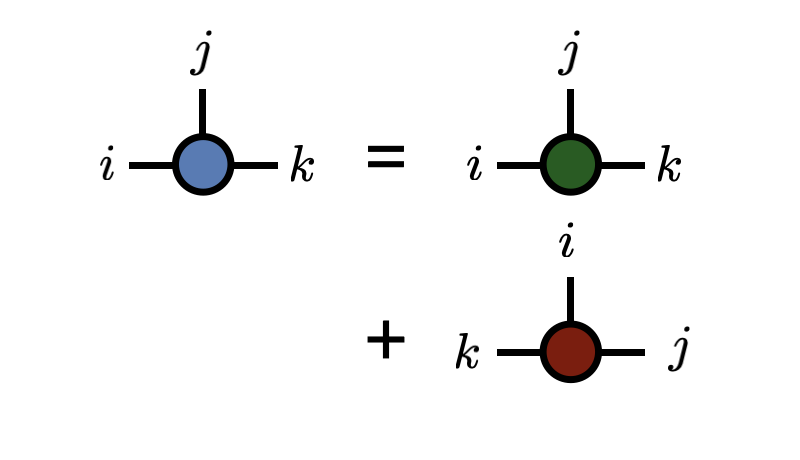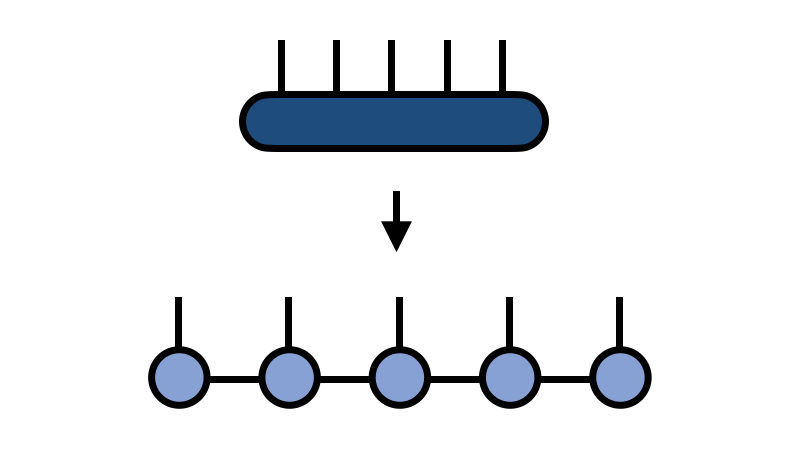Constructing an ITensor``````i = Index(3,"index_i")
j = Index(2,"index_j")
k = Index(4,"index_k")

T = ITensor(i,j,k)``````

Setting an element of an ITensor``````T = ITensor(i,j,k)

T[j=>1,k=>3,i=>2] = -3.2``````

Adding ITensors (note how indices can be in different order)``````A = randomITensor(i,j,k)
B = randomITensor(k,i,j)

C = A + B``````

Making an MPS from an ITensor``````T = randomITensor(i,j,k,l,m)
M = MPS(T,(i,j,k,l,m); maxdim=10)``````

For more code examples, see the documentation.